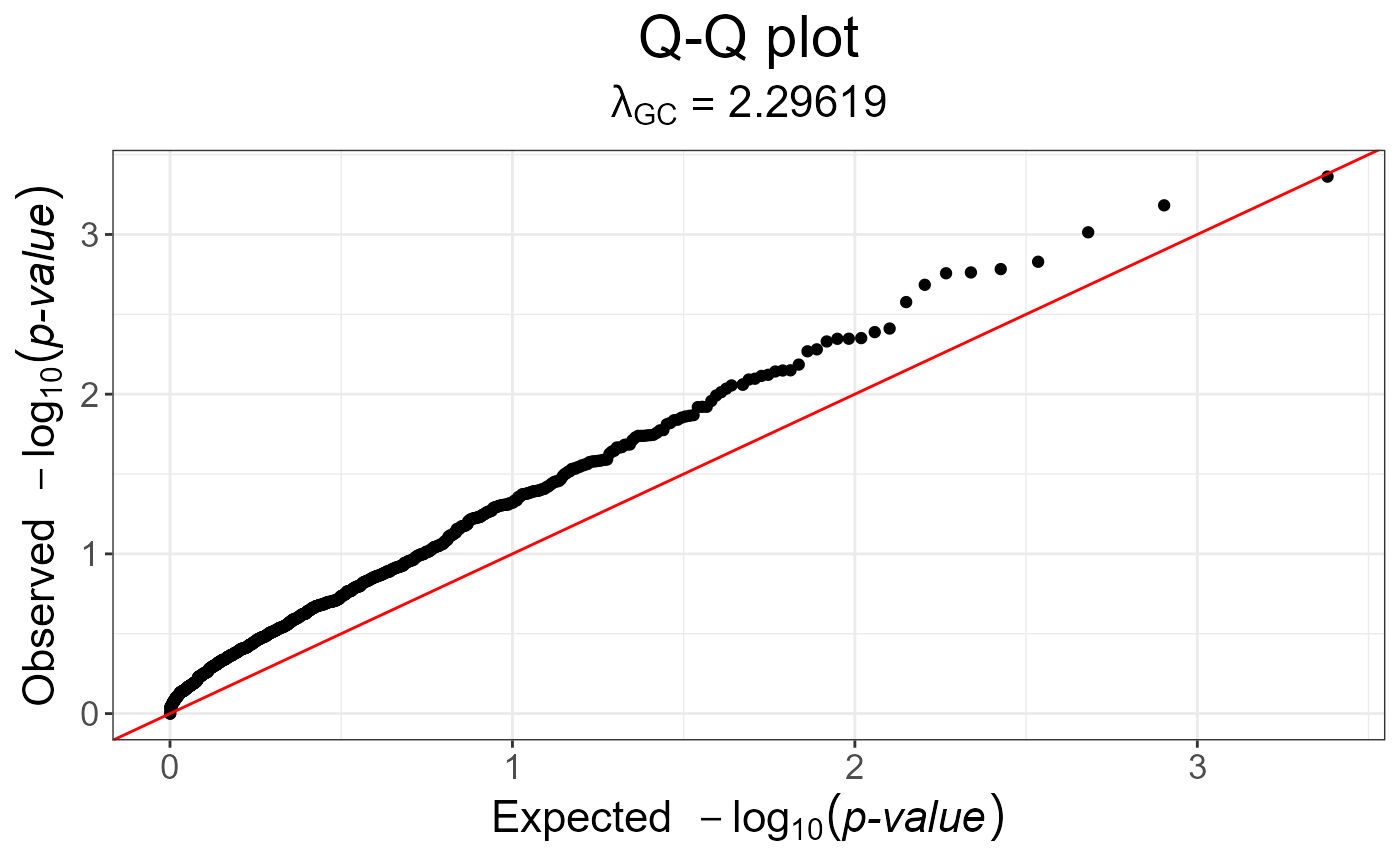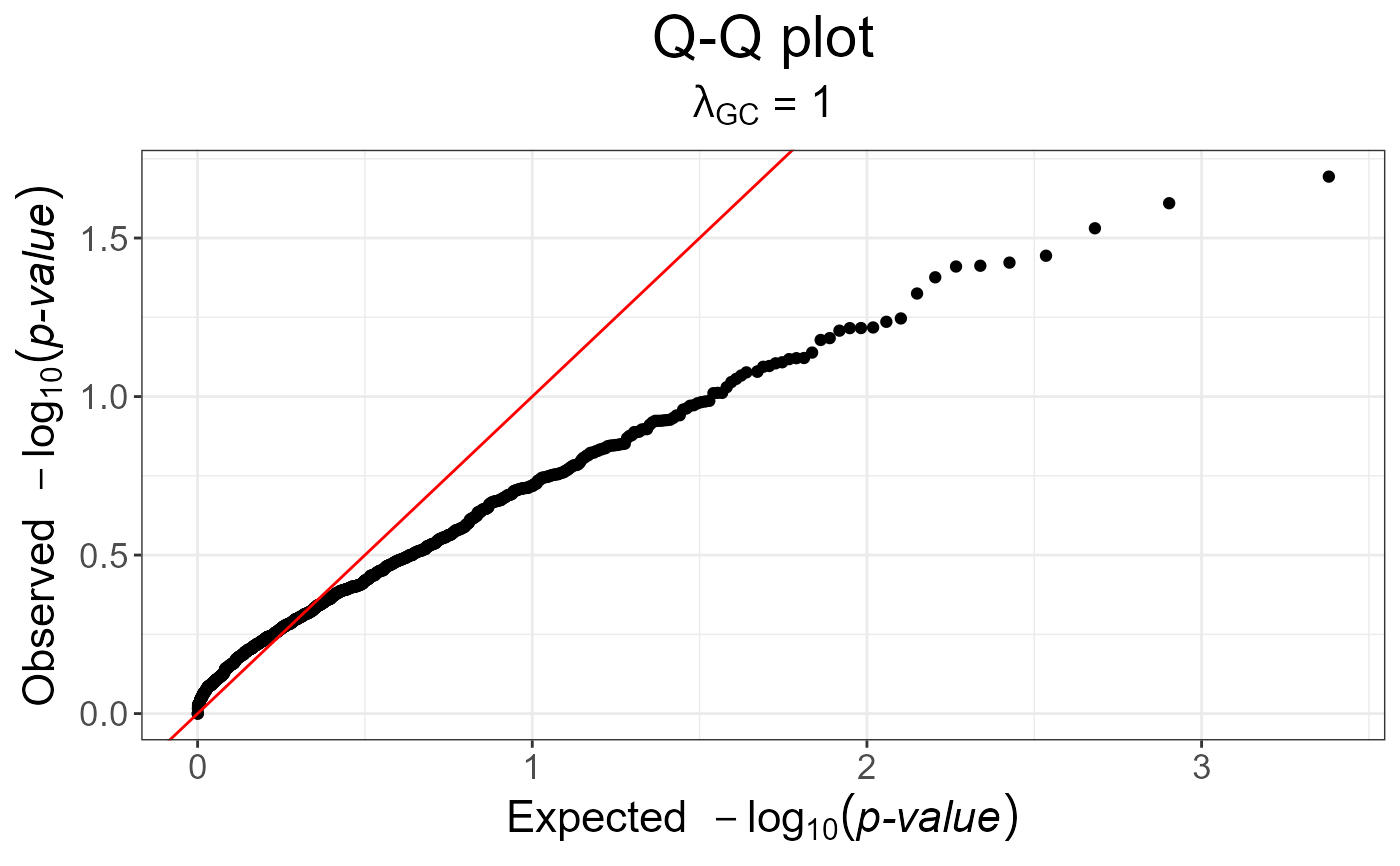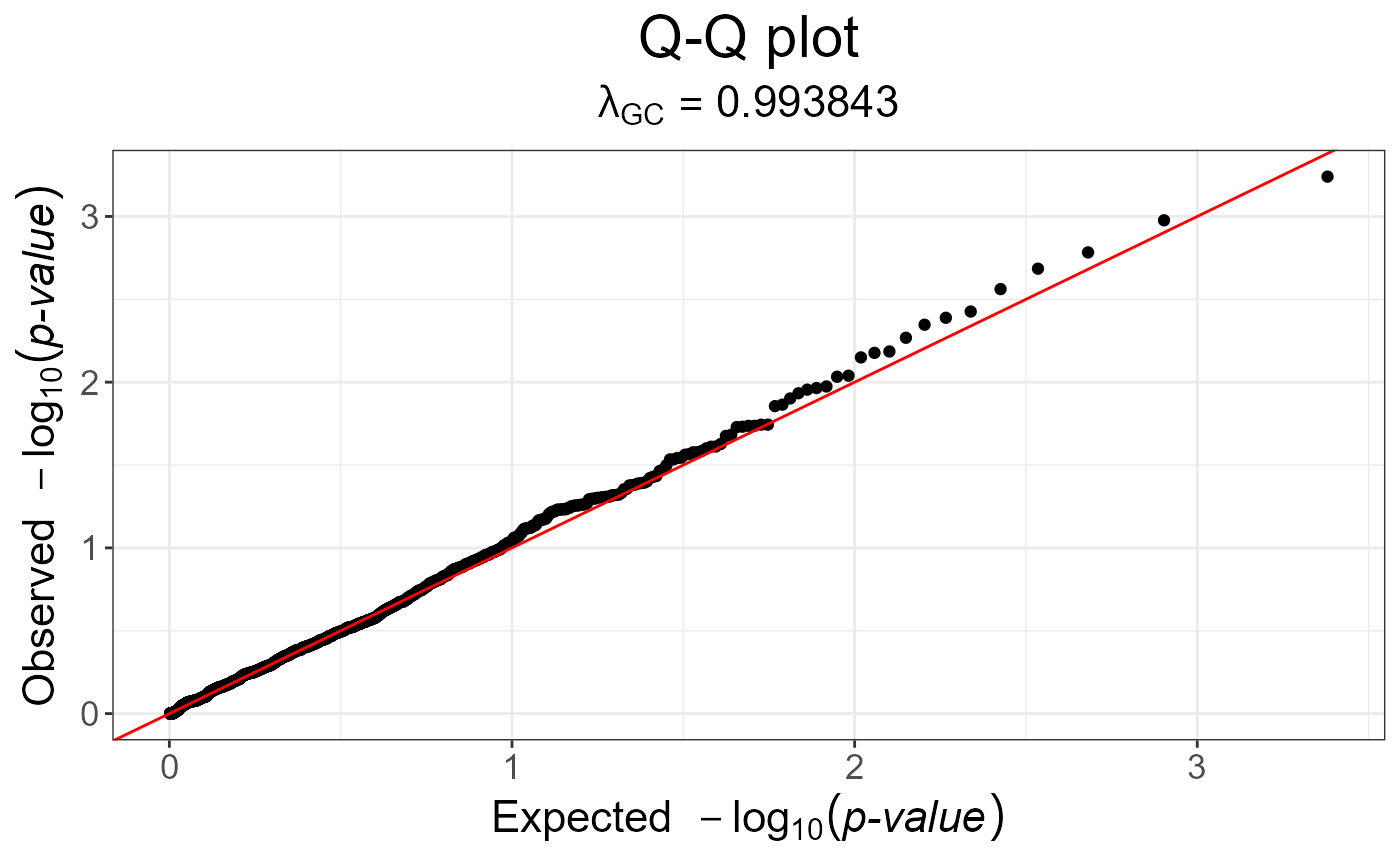Compute the MAX3 statistic, which tests for three genetic models (additive, recessive and dominant).

snp_MAX3(Gna, y01.train, ind.train = rows_along(Gna), val = c(0, 0.5, 1))

Gna

A FBM.code256 (typically <bigSNP>$genotypes). You can have missing values in these data. y01.train Vector of responses, corresponding to ind.train. Must be only 0s and 1s. ind.train An optional vector of the row indices that are used, for the training part. If not specified, all rows are used. Don't use negative indices. val Computing $$\smash{\displaystyle\max_{x \in val}}~Z_{CATT}^2(x)$$. • Default is c(0, 0.5, 1) and corresponds to the MAX3 statistic. • Only c(0, 1) corresponds to MAX2. • And only 0.5 corresponds to the Armitage trend test. • Finally, seq(0, 1, length.out = L) corresponds to MAXL. ## Value An object of classes mhtest and data.frame returning one score by SNP. See methods(class = "mhtest"). ## Details P-values associated with returned scores are in fact the minimum of the p-values of each test separately. Thus, they are biased downward. ## Examples set.seed(1) # constructing a fake genotype big.matrix N <- 50; M <- 1200 fake <- snp_fake(N, M) G <- fake$genotypes
G[] <- sample(as.raw(0:3), size = length(G), replace = TRUE)
G[1:8, 1:10]
#>      [,1] [,2] [,3] [,4] [,5] [,6] [,7] [,8] [,9] [,10]
#> [1,]    0    0   NA    0    2    1    0   NA   NA     2
#> [2,]   NA    0   NA    2   NA    2    2    2    2     1
#> [3,]    2    2    2    2    1    2    1    0    2     0
#> [4,]   NA    1    0   NA    0    2    2    0    0     2
#> [5,]    0    0   NA   NA    2   NA    2    2   NA    NA
#> [6,]    1    0   NA    2    2   NA    1    2    2     0
#> [7,]    2   NA    1    1    1   NA    1    2    1    NA
#> [8,]    2    2    1    0    1   NA   NA    2    0     1

# Specify case/control phenotypes
fake$fam$affection <- rep(1:2, each = N / 2)

# Get MAX3 statistics
y01 <- fake$fam$affection - 1
str(test <- snp_MAX3(fake$genotypes, y01.train = y01)) #> Classes 'mhtest' and 'data.frame': 1200 obs. of 1 variable: #>$ score: num  1.7124 0.0178 2.7611 0.1309 0.4396 ...
#>  - attr(*, "transfo")=function (x)
#>  - attr(*, "predict")=function (xtr)
# p-values are not well calibrated
snp_qq(test)# genomic control is not of much help
snp_qq(snp_gc(test))# Armitage trend test (well calibrated because only one test)
test2 <- snp_MAX3(fake\$genotypes, y01.train = y01, val = 0.5)
snp_qq(test2)